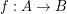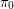direct product

A construction of direct product in the category of continuous maps between endo-funcoids ★★★

Author(s): Porton

Consider the category of (proximally) continuous maps (entirely defined monovalued functions) between endo-funcoids.

Remind from my book that morphismsof this category are defined by the formula(here and below by abuse of notation I equate functions with corresponding principal funcoids).

Letare endofuncoids,

We define(hereandare cartesian projections).

Conjecture   The above defines categorical direct product (in the above mentioned category, with products of morphisms the same as in Set).

Keywords: categorical product; direct product